Courses

# Test: Relation And Function (Competition Level) - 2

## 30 Questions MCQ Test Mathematics for JEE Mains | Test: Relation And Function (Competition Level) - 2

Description
This mock test of Test: Relation And Function (Competition Level) - 2 for JEE helps you for every JEE entrance exam. This contains 30 Multiple Choice Questions for JEE Test: Relation And Function (Competition Level) - 2 (mcq) to study with solutions a complete question bank. The solved questions answers in this Test: Relation And Function (Competition Level) - 2 quiz give you a good mix of easy questions and tough questions. JEE students definitely take this Test: Relation And Function (Competition Level) - 2 exercise for a better result in the exam. You can find other Test: Relation And Function (Competition Level) - 2 extra questions, long questions & short questions for JEE on EduRev as well by searching above.
QUESTION: 1

### Let  A = {1, 2, 3} and let R = {(1, 1), (2, 2), (3, 3), (1, 3), (3, 2), (1, 2)}. Then R is

Solution:

R is reflexive and transitive but not symmetric

QUESTION: 2

### Let A {a, b, c} and let R = {(a, a)(a, b), (b, a)}. Then, R is

Solution:

R is symmetric and transitive but not reflexive

QUESTION: 3

### Let A = {1, 2, 3} then total number of element in A x A is

Solution:
QUESTION: 4

Let S be the set of all straight lines in a plane. Let R be a relation on S defined by a R b ⇔ a ⊥ b. then, R is

Solution:

a ⊥ a is not true. So, R is not reflexive
a ⊥ b and b ⊥ c does not imply a ⊥ c. So, R is not transitive
But, a ⊥ b ⇒ b ⊥ a is always true.

QUESTION: 5

Let S be the set of all real numbers and let R be a relation on S, defined by a R b ⇔ |a – b| < 1. Then, R is

Solution:

(i) |a – a| = 0 < 1 is always true
(ii) a R b ⇒ |a – b| < 1 ⇒ |-(a – b)| < ⇒ |b – a| < 1 ⇒ b R a.
(iii) 2R 1 and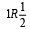But, 2 is not related to 1/2. So, R is not transitive.

QUESTION: 6

Domain of f (x)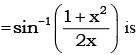Solution:

Domain of sin -1x is [-1,1]

QUESTION: 7

Let R be the relation in the set N given by R = {(a, b): a = b – 2, b > 6}. Choose the correct answer.

Solution:

R = {(a, b): a = b − 2, b > 6}
Now, since b > 6, (2, 4) ∉ R
Also, as 3 ≠ 8 − 2, (3, 8) ∉ R
And, as 8 ≠ 7 − 2
∴ (8, 7) ∉ R
Now, consider (6, 8).
We have 8 > 6 and also, 6 = 8 − 2.
∴ (6, 8) ∈ R

QUESTION: 8

Let R = {(3, 3), (6, 6), (9, 9), (3,6), (3, 9), (9, 12), (3,12), (6, 12), (12, 12)}, be a relation on the set A = {3, 6, 9, 12} Then the relation is

Solution:

R is reflexive
∴ (3, 3), (6,6), (9, 9), (12, 12) ∈ R
again ∴ (6, 12) ∈ R but (12, 6) ∉ R ⇒ R is not symmetric
R is transitive
[∴ (3, 6) ∈ R, (6, 12) ∈ R, (3, 12) ∈ R others are clear

QUESTION: 9

If f(x) = (a – xn)1/n.  then f(f(x)) =

Solution:

f(x) = (a – xn)1/n = y

QUESTION: 10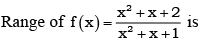Solution:
QUESTION: 11

f: N → N : f(x) = 2x is

Solution:

2x = 3 ⇒ x = 3/2 ∉ N. So, f is in to many-one and into
many-one and into

QUESTION: 12

f: R → R : f(x) = x2 is

Solution:

Given, function f : R→R such that f(x) = x2
Let A and B be two sets of real numbers.
Let x1, x2 ∈ A such that f(x1) = f(x2).
⇒1 + (x1)2 = 1 + (x2)2
​⇒ (x1)2 − (x2)2 = 0
⇒(x1 − x2)(x1 + x2)=0
⇒ x1 = ± x2
Thus f(x1) = f(x2) does not imply that x1 = x2.
For instance, f(1) = f(−1) = 1, i.e. , two elements (1, -1) of A have the same image in B. So, f is many-one function.

QUESTION: 13

f : R → R : f(x) = x3 is

Solution: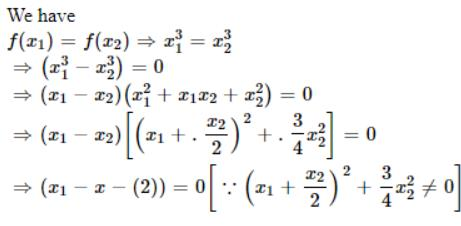⇒ x2 = x2

∴ f is one-one

Let y∈R and let y=x3. then x=y ∈ R

Thus for each y in the codomain R there exists y1/3 in R such that

f(y13)=(y13)3=y

∴ f is onto.

Hence f is one -one onto.

QUESTION: 14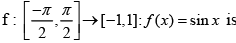Solution:

f(x) = sinx
f(x) = sinx is a one-one function.
codomain = range
therefore, f(x) = sinx is an onto function.

QUESTION: 15

f : R → R : f(x) = cos x is

Solution:

cos(2π - θ) = cosq ⇒ f is many-one.
Range (f) = [-1,1] ⊂ R ⇒ f is in to.

QUESTION: 16

The domain of the function f = {(1, 3), (3, 5), (2, 6)} is

Solution:

Domain = {1, 3, 2}

QUESTION: 17

Let f(x) = x - 1/x +1 , x ≠ -1, then f-1 (x) is

Solution:
QUESTION: 18

If f(x) = x/x -1 , x ≠ 1, then f-1 (x) is

Solution:
QUESTION: 19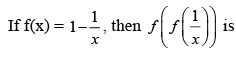Solution:
QUESTION: 20

If f(x) = cos (log x), then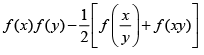has the value

Solution: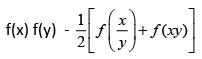= cos (log x) cos (cos y)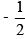[cos(log(x/y))+cos(log(xy))]
= cos (log x) cos (log y)[cos(log x - log y)+cos(log x +log y)]
= cos (log x) cos (log y)[2 cos(log  x)cos(log y)] = 0

QUESTION: 21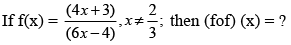Solution: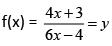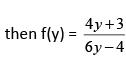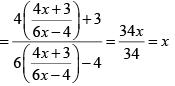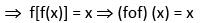QUESTION: 22

If f(x) =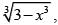then (fof) (x) = ?

Solution:

(fof) (x) = f[f(x)] – {(3 – x3)1/3} = f(y)  where y = (3 – x3)1/3
= (3 – y3)1/3 = [3- (3 – x3)]1/3 = (x3)1/3 = x

QUESTION: 23

If f(x) = x2 – 3x + 2, then (fof) (x) = ?

Solution:

(fof) (x) = f[f(x)] = f(x2 - 3x + 2)2 - 3(x2 – 3x + 2)
= y2 – 3y + 2 = (x2 – 3x + 2)2 – 3(x2 – 3x + 2) + 2 =  (x4 – 6x3 + 10x2 – 3x)

QUESTION: 24

Let S = {1, 2, 3}. The function f : S → S defined as below have inverse for

Solution:

Since f(2) = f(3) = 1, then inverse does not exists
Inverse exist for (C) f-1 = {(3, 1), (2, 3), (1, 2)}.

QUESTION: 25

The relation R defined on the set N of natural numbers by xRy ⇔2x2 - 3xy + y2 = 0 is

Solution:

(i) xRx ⇔ 2x2 - 3x.x + x2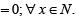∴ R is reflexive
(ii) For x = 1, y= 2; 2x2 - 3xy + y2 = 0
∴ 1 R 2 but 2.22 - 3.2.1 + 12 = 3 ≠ 0.
So, 2 is not R-related to 1.
∴ R is not symmetric.

QUESTION: 26

Let R = {(3, 3), (6, 6), (9, 9), (12, 12), (6, 12), (3, 9), (3, 12), (3, 6) be a relation on the set A = {3, 6, 9, 12}. The relation is

Solution:

Since, (3, 3), (6, 6), (9, 9), (12, 12) ∈ R ⇒ R is reflexive relation.
Now, (6, 12)∉R but (12, 6) ∉ R  ⇒ R is not a symmetric relation.
Also, (3, 6),(6, 12) ∈ R ⇒ (3, 12) ∈ R
⇒ R is transitive relation.

QUESTION: 27

Let R be a relation on the set A of ordered pairs of positive integers defined by (x, y)R(u, v) if and only if xv = yu, then

Solution:

Clearly, (x, y)R(x, y),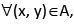since xy = yx. This shows that R is reflexive.
Further, (x, y)R(u, v) ⇒ xv = yu ⇒ uy vx and hence (u, v)R(x, y). This shows that R is symmetric.
Similarly, (x, y)R(u, v) and (u, v)R(a, b) ⇒ xv = yu and ub = va ⇒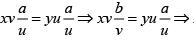xb = ya and hence (x, y)R(a, b). Thus, R is transitive. Thus, R is an equivalent relation.

QUESTION: 28

If R be a relation defined as aRb iff |a -b|> 0, then the relation is

Solution:

Since, R is a defined as aRb iff |a – b| > 0
For reflexive aRa iff |a – a| > 0
Which is not true, So, R is not reflexive
For symmetric aRb iff |a – b| > 0
Now bRa iff |b – a| > 0
⇒ |a – b| > 0  ⇒ ArB
Thus, R is symmetric.
For transitive aRb iff |a – b| > 0
bRc iff |b – c| > 0
⇒ |a – b + b – c| > 0
⇒ |a – c| > 0 ⇒ | c – a| > 0 ⇒ aRc
∴ R is transitive

QUESTION: 29

If R is an equivalence relation of a set A, then R-1 is

Solution:

If R is an equivalence relation, then R-1 is also an equivalence relation.

QUESTION: 30

Let r be a relation from R (set of real numbers) to R defined by r = {(a, b)|a, b∈R and a - b + √3 is an irrational number}. The relation r is

Solution:

Given, r = {(a, b)| a, b∈R and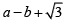as an irrational number
(i) Reflexive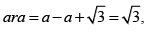which is irrational number.
(ii) Symmetric
Now,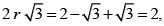which is not an irrational.
Also,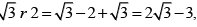which is an irrational.
Which is not symmetric.
(iii) Transitive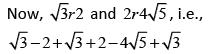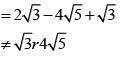∴ It is not transitive.